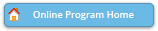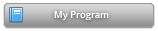#### Abstract Details

 Activity Number: 254 - Contributed Poster Presentations: Section on Statistical Learning and Data Science Type: Contributed Date/Time: Monday, July 30, 2018 : 2:00 PM to 3:50 PM Sponsor: Section on Statistical Learning and Data Science Abstract #329203 Title: Multivariate Zero-Inflated Poisson Regression Author(s): Yang Wang* Companies: University of Alabama Keywords: Multivariate Poisson regression; Multivariate zero-inflated Poisson regression; EM algorithm; MLE Abstract: In this report, we develop a procedure to analyze the relationship between the observed multi-dimensional counts and a set of explanatory variables. The counts follow a multivariate Poisson distribution or a multivariate zero-inflated Poisson distribution. Maximum likelihood estimates (MLE) for the model parameters are obtained by the Newton-Raphson (NR) iteration and the expectation-maximization (EM) algorithm, respectively. In Newton-Raphson method, the fi rst and second derivatives of the log-likelihood function are derived to carry out the numerical evaluation. Formulas using EM algorithm are also introduced. A comparison of the estimation performance is made from simulation studies.

Authors who are presenting talks have a * after their name.